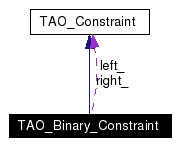# TAO_Binary_Constraint Class Reference

TAO_Binary_Constraint represents an operation with left and right operands. More...

`#include <Constraint_Nodes.h>`

Inheritance diagram for TAO_Binary_Constraint:[legend]
Collaboration diagram for TAO_Binary_Constraint:[legend]
List of all members.

## Public Member Functions

TAO_Binary_Constraint (TAO_Expression_Type op_type, TAO_Constraint *left, TAO_Constraint *right)
virtual int accept (TAO_Constraint_Visitor *visitor)
virtual ~TAO_Binary_Constraint (void)
virtual TAO_Expression_Type expr_type (void) const
Return the expression type represented by this node.
TAO_Constraintleft_operand (void) const
Return the left operand of the binary expression.
TAO_Constraintright_operand (void) const
Return the right operand of the binary expression.

## Static Public Member Functions

int visit_or (TAO_Constraint_Visitor *, TAO_Binary_Constraint *)
int visit_and (TAO_Constraint_Visitor *, TAO_Binary_Constraint *)
int visit_less_than (TAO_Constraint_Visitor *, TAO_Binary_Constraint *)
int visit_less_than_equal (TAO_Constraint_Visitor *, TAO_Binary_Constraint *)
int visit_greater_than (TAO_Constraint_Visitor *, TAO_Binary_Constraint *)
int visit_greater_than_equal (TAO_Constraint_Visitor *, TAO_Binary_Constraint *)
int visit_equal (TAO_Constraint_Visitor *, TAO_Binary_Constraint *)
int visit_not_equal (TAO_Constraint_Visitor *, TAO_Binary_Constraint *)
int visit_add (TAO_Constraint_Visitor *, TAO_Binary_Constraint *)
int visit_sub (TAO_Constraint_Visitor *, TAO_Binary_Constraint *)
int visit_mult (TAO_Constraint_Visitor *, TAO_Binary_Constraint *)
int visit_div (TAO_Constraint_Visitor *, TAO_Binary_Constraint *)
int visit_twiddle (TAO_Constraint_Visitor *, TAO_Binary_Constraint *)
int visit_in (TAO_Constraint_Visitor *, TAO_Binary_Constraint *)

## Private Member Functions

TAO_Binary_Constraint (const TAO_Binary_Constraint &)
TAO_Binary_Constraintoperator= (const TAO_Binary_Constraint &)

## Private Attributes

TAO_Expression_Type op_
The operator type.
TAO_Constraintleft_
The operands of the expression.
TAO_Constraintright_

## Detailed Description

TAO_Binary_Constraint represents an operation with left and right operands.

## Constructor & Destructor Documentation

 TAO_Binary_Constraint::TAO_Binary_Constraint ( TAO_Expression_Type op_type, TAO_Constraint * left, TAO_Constraint * right )

 TAO_Binary_Constraint::~TAO_Binary_Constraint ( void ) ` [virtual]`

 TAO_Binary_Constraint::TAO_Binary_Constraint ( const TAO_Binary_Constraint & ) ` [private]`

## Member Function Documentation

 int TAO_Binary_Constraint::accept ( TAO_Constraint_Visitor * visitor ) ` [virtual]`
 Implementing the pattern of double dispatching, each subclass of TAO_Constraint will call back on an InterpreterVisitor the method to handle a node of its ExpressionType. Implements TAO_Constraint.

 virtual TAO_Expression_Type TAO_Binary_Constraint::expr_type ( void ) const` [inline, virtual]`
 Return the expression type represented by this node. Implements TAO_Constraint.

 TAO_Constraint * TAO_Binary_Constraint::left_operand ( void ) const
 Return the left operand of the binary expression.

 TAO_Binary_Constraint& TAO_Binary_Constraint::operator= ( const TAO_Binary_Constraint & ) ` [private]`

 TAO_Constraint * TAO_Binary_Constraint::right_operand ( void ) const
 Return the right operand of the binary expression.

 int TAO_Binary_Constraint::visit_add ( TAO_Constraint_Visitor * , TAO_Binary_Constraint * ) ` [static]`

 int TAO_Binary_Constraint::visit_and ( TAO_Constraint_Visitor * , TAO_Binary_Constraint * ) ` [static]`

 int TAO_Binary_Constraint::visit_div ( TAO_Constraint_Visitor * , TAO_Binary_Constraint * ) ` [static]`

 int TAO_Binary_Constraint::visit_equal ( TAO_Constraint_Visitor * , TAO_Binary_Constraint * ) ` [static]`

 int TAO_Binary_Constraint::visit_greater_than ( TAO_Constraint_Visitor * , TAO_Binary_Constraint * ) ` [static]`

 int TAO_Binary_Constraint::visit_greater_than_equal ( TAO_Constraint_Visitor * , TAO_Binary_Constraint * ) ` [static]`

 int TAO_Binary_Constraint::visit_in ( TAO_Constraint_Visitor * , TAO_Binary_Constraint * ) ` [static]`

 int TAO_Binary_Constraint::visit_less_than ( TAO_Constraint_Visitor * , TAO_Binary_Constraint * ) ` [static]`

 int TAO_Binary_Constraint::visit_less_than_equal ( TAO_Constraint_Visitor * , TAO_Binary_Constraint * ) ` [static]`

 int TAO_Binary_Constraint::visit_mult ( TAO_Constraint_Visitor * , TAO_Binary_Constraint * ) ` [static]`

 int TAO_Binary_Constraint::visit_not_equal ( TAO_Constraint_Visitor * , TAO_Binary_Constraint * ) ` [static]`

 int TAO_Binary_Constraint::visit_or ( TAO_Constraint_Visitor * , TAO_Binary_Constraint * ) ` [static]`

 int TAO_Binary_Constraint::visit_sub ( TAO_Constraint_Visitor * , TAO_Binary_Constraint * ) ` [static]`

 int TAO_Binary_Constraint::visit_twiddle ( TAO_Constraint_Visitor * , TAO_Binary_Constraint * ) ` [static]`

## Member Data Documentation

 TAO_Constraint* TAO_Binary_Constraint::left_` [private]`
 The operands of the expression.

 TAO_Expression_Type TAO_Binary_Constraint::op_` [private]`
 The operator type.

 TAO_Constraint* TAO_Binary_Constraint::right_` [private]`

The documentation for this class was generated from the following files:
Generated on Thu Feb 10 21:03:41 2005 for TAO_CosTrader by1.3.9.1